# HBSE 8th Class Maths Solutions Chapter 11 Mensuration Ex 11.2

Haryana State Board HBSE 8th Class Maths Solutions Chapter 11 Mensuration Ex 11.2 Textbook Exercise Questions and Answers.

## Haryana Board 8th Class Maths Solutions Chapter 11 Mensuration Exercise 11.2

Question 1.
The shape of the top surface of a table is a trapezium. Find its area if its parallel sides are 1 m and 1.2 m and perpendicular distance between them is 0.8 m.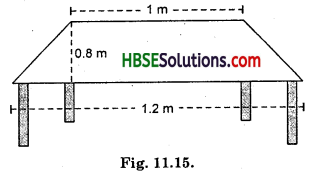Solution:
Area of trapezium shape
= $$\frac{1}{2}$$(b1 + b2) × h
= $$\frac{1}{2}$$(1.2 + 1) × 0.8 2
= $$\frac{1}{2}$$ × 2.2 × 0.8 = 0.88 m2.

Question 2.
The area of a trapezium is 34 cm2 and the length of one of the parallel sides is 10 cm and its height is 4 cm. Find the length of the other parallel side.
Solution:
Area of trapezium
= $$\frac{1}{2}$$(b1 + b2) × h
34 = $$\frac{1}{2}$$(10.4 + b2) × 4
34 = (10.4 + b2) × 2
34 = 20.8 + 2b2
34 – 20.8 = 2b2
13.2 = 2b2
$$\frac{13.2}{2}$$ = b2
6.1 = b2
Hence another parallel side = 6.6 cm.Question 3.
Length of the fence of a trapezium shaped field ABCD is 120 m. If BC = 48 m, CD = 17 m and AD = 40 m, find the area of this field. Side AB is perpendicular to the parallel sides AD and BC.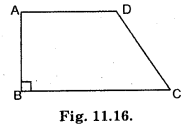Solution:
Perimeter of ABCD
= AB + BC + CD + 40
120 = AB + 48+ 17 + 40
= AB + 105
or 120 – 105 = AB
or 15 = AB
∴ AB = 15 cm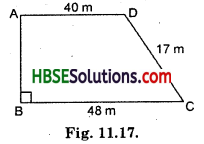Area of trapezium ABCD
= $$\frac{1}{2}$$(b1 + b2) × k
= $$\frac{1}{2}$$ × (48 + 40) × 15
= $$\frac{1}{2}$$ × 88 × 15 = 660 m2.

Question 4.
The diagonal of a quadrilateral shaped field is 24 m and the perpendiculars dropped on it from the remaining opposite vertices are 8 m and 13 m. Find the area of the field.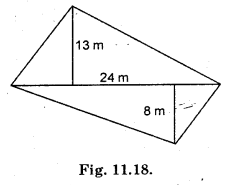Solution:
= Area of ABC + Area of ADC
= $$\frac{1}{2}$$ × AC × OD + $$\frac{1}{2}$$ × AC × BP
= $$\frac{1}{2}$$ × 24 × 13 + $$\frac{1}{2}$$ × 24 × 8 2 2
= 12 × 13 + 12 × 8 = 12(13 + 8)
= 12 × 21 = 252 m2.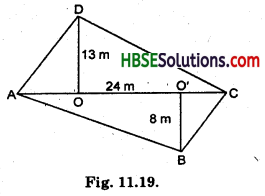Question 5.
The diagonals of a rhombus are 7.5 cm and 12 cm. Find its area.
Solution:
Area of rhombus = $$\frac{1}{2}$$ × d1 × d2
= $$\frac{1}{2}$$ × 7.5 × 12
= 7.5 × 6 = 45.0
= 45 cm2.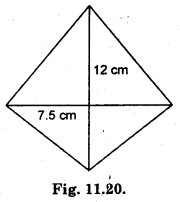Question 6.
Find the area of a rhombus whose side is 6 cm and whose altitude is 4 cm. If one of its diagonals is 8 cm long, find the length of the other diagonal.
Solution:
Area of DADO = $$\frac{1}{2}$$ × AO × OD
= $$\frac{1}{2}$$ × 4 × 4 = 8 cm2
Hence, Area of 4 triangle = 4 × 8 = 32 cm2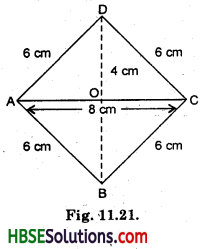Now, Area of trapezium = $$\frac{1}{2}$$ × d1 × d2
32 cm2 = $$\frac{1}{2}$$ × 8 × d2
32 cm2 = 4d2
$$\frac{32 \mathrm{~cm}^{2}}{4 \mathrm{~cm}}$$= d2
8 cm = d2
Hence, the length of other diagonal = 8 cm.Question 7.
The floor of a building consists of 3000 tiles which are rhombus shaped and each of its diagonals are 45 cm and 30 cm in length. Find the total cost of polishing the floor, if the cost per m2 is Rs. 4.
Solution:
Area of rhombus of each tiles
= $$\frac{1}{2}$$ × d1 × d2
= $$\frac{1}{2}$$ × 45 × 30
= 675 cm2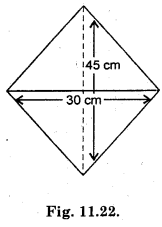Area of 3000 tiles = 3000 × 675
= $$\frac{2025000 \mathrm{~cm}^{2}}{100 \mathrm{~cm} \times 100 \mathrm{~cm}}$$
= 202.5 m2
Thecost of 3000 tiles = 202.5 × 4
= Rs. 810.0
= Rs.810.

Question 8.
Mohan wants to buy a trapezium shaped field. Its side along the river is parallel to and twice the side along the road. If the area of this Held is 10500 m2 and the perpendicular distance between the two parallel sides is 100 m, find the length of the side along the river.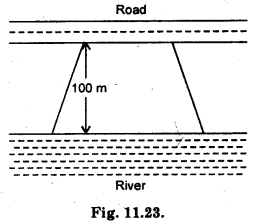Solution:
Let the one side = x m = b1
and other side = 2x m = b2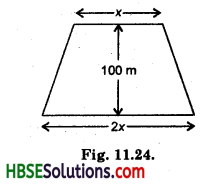Area of trapezium = $$\frac{1}{2}$$(b1 + b2) × h
= $$\frac{1}{2}$$(x + 2x) × 100
= $$\frac{1}{2}$$ × 3x + 100
or 10500m2 = 150x
or $$\frac{10500}{150}$$ = x
70 = x
∴ x = 70 cm
Hence One side = 70 cm
and Another parallel side = 2 × 70 = 140 m.Question 9.
Top surface of a raised platform is in the shape of a regular octagon as shown in the figure. Find the area of the octagonal surface.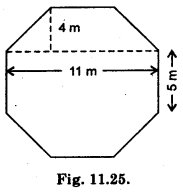Solution:
Area of another trapezium
= $$\frac{1}{2}$$(d1 + d2) × h
= $$\frac{1}{2}$$(11 + 5) × 4
= $$\frac{1}{2}$$ × 16 × 4 = 32 m2
Area of rectangle
= l × b = 11 m × 5 m = 5m2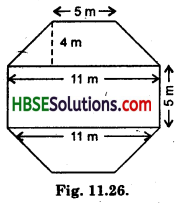= $$\frac{1}{2}$$(d1 + d2) × h
= $$\frac{1}{2}$$(11 + 5) × 4 = 32 m2
∴ Area of the octagonal surface = Area of one trapezium + Rectangle + Area of another trapezium
= 32 m2 + 55 m2 + 32 m2 =119 m2.

Question 10.
There is a pentagonal shaped park as shown in the figure.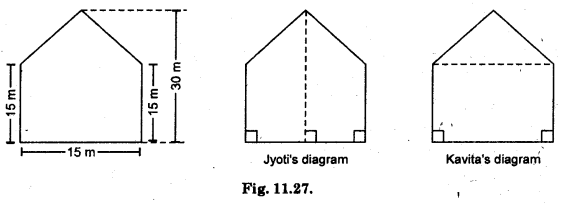For finding its area Jyoti and Kavita divided it in two different ways.
Find the area of this park using both ways. Can you suggest some other way of finding its area ?
Solution:
Jyoti’ diagram :
= $$\frac{1}{2}$$(b1 + b2) + h
= $$\frac{1}{2}$$(15 + 30) × 7.5
= $$\frac{1}{2}$$ × 45 × 7.5
= 168.75 cm2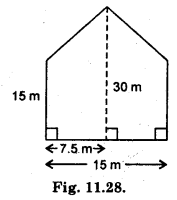Hence, Area of two trapezium
= 2 × 168.75 = 237.50 cm2
Kavita’s diagram:
= Area of Kavita’s diagram
= $$\frac{1}{2}$$ × 15 × 15 + (15)2
[∵ 30 – 15 = 15]
= $$\frac{225}{2}$$ + $$\frac{225}{1}$$ = $$\frac{225+50}{2}$$
= $$\frac{675}{2}$$
= 337.5 cm2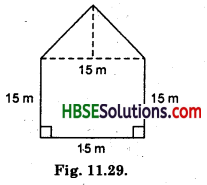Question 11.
Diagram of the adjacent picture frame has outer dimensions = 24 cm × 28 cm and inner dimensions 16 cm × 20 cm. Find the area of each section of the frame, if the width of each section is same.
Solution:
Area of outer frame
= l × b
= 28 × 24
= 672 cm2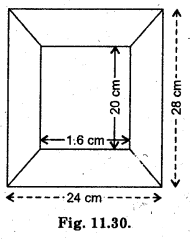Area of inner frame
= l × b = 16 × 20 = 320 cm2
Hence, area of remaining frame
= 672 – 320 = 352 cm2
Now, area of each section
= $$\frac{352}{4}$$ = 88 cm2